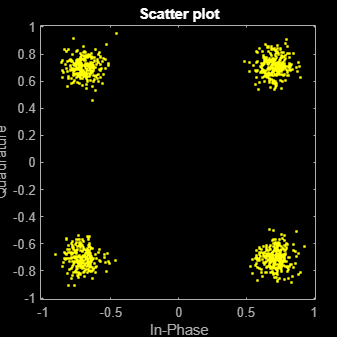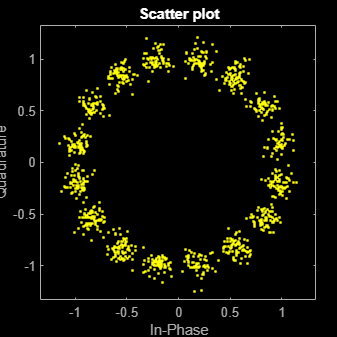Documentation

This is machine translation

Mouseover text to see original. Click the button below to return to the English version of the page.

pskmod

Phase shift keying modulation

Syntax

``y = pskmod(x,M)``
``y = pskmod(x,M,ini_phase)``
``y = pskmod(x,M,ini_phase,symorder)``

Description

````y = pskmod(x,M)` modulates the input signal, `x`, using phase shift keying (PSK) with modulation order `M`.```

example

````y = pskmod(x,M,ini_phase)` specifies the initial phase of the PSK-modulated signal.```
````y = pskmod(x,M,ini_phase,symorder)` specifies the symbol order of the PSK-modulated signal.```

Examples

collapse all

Modulate and plot the constellations of QPSK and 16-PSK signals.

QPSK

Set the modulation order to 4.

`M = 4;`

Generate random data symbols.

`data = randi([0 M-1],1000,1);`

Modulate the data symbols.

`txSig = pskmod(data,M,pi/M);`

Pass the signal through white noise and plot its constellation.

```rxSig = awgn(txSig,20); scatterplot(rxSig)```16-PSK

Change the modulation order from 4 to 16.

`M = 16;`

Generate random data symbols.

`data = randi([0 M-1],1000,1);`

Modulate the data symbols.

`txSig = pskmod(data,M,pi/M);`

Pass the signal through white noise and plot its constellation.

```rxSig = awgn(txSig,20); scatterplot(rxSig)```Generate random symbols.

`dataIn = randi([0 3],1000,1);`

QPSK modulate the data.

`txSig = pskmod(dataIn,4,pi/4);`

Pass the signal through an AWGN channel.

`rxSig = awgn(txSig,10);`

Demodulate the received signal and compute the number of symbol errors.

```dataOut = pskdemod(rxSig,4,pi/4); numErrs = symerr(dataIn,dataOut)```
```numErrs = 2 ```

Input Arguments

collapse all

Input signal, specified as a vector or matrix of positive integers. The elements of `x` must have values in the range of [0, `M` – 1].

Example: `randi([0 3],100,1)`

Data Types: `double` | `single`

Modulation order, specified as an integer power of two.

Example: `2` | `4` | `16`

Data Types: `double` | `single`

Initial phase of the PSK modulation, specified in radians as a real scalar.

If you specify `ini_phase` as empty, then `pskmod` uses an initial phase of 0.

Example: `pi/4`

Data Types: `double` | `single`

Symbol order, specified as `'bin'` or `'gray'`. This argument specifies how the function assigns binary vectors to corresponding integers.

• If `symorder` is `'bin'`, the function uses a natural binary-coded ordering.

• If `symorder` is `'gray'`, the function uses a Gray-coded ordering.

Data Types: `char`

Output Arguments

collapse all

Complex baseband representation of a PSK-modulated signal, returned as vector or matrix. The columns of `y` represent independent channels.

Data Types: `double` | `single`
Complex Number Support: Yes